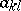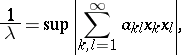# Fredholm eigenvalue of a Jordan curve

Let $C$ be a smooth Jordan curve (of class $C ^ { 3 }$) in the complex $z = ( x + i y )$-plane, $G$ its interior, $G ^ { * }$ its exterior. Then, let

\begin{equation*} K ( x , t ) = - \frac { 1 } { \pi } \frac { \partial } { \partial n _ { t } } \operatorname { log } | z - t | , z , t \in C, \end{equation*}

be the corresponding classical Neumann kernel with $n_t$ the interior normal. The Fredholm eigenvalue $\lambda$ of $C$ is the smallest eigenvalue $> 1$ of this kernel.

This eigenvalue plays an important role for the speed of the successive approximation solution of several problems, such as the integral equation with this kernel corresponding to the Dirichlet problem and several integral equations to construct the conformal Riemann mapping function of $G$ [a1].

For arbitrary Jordan curves $C$, there is the following characterization of the Fredholm eigenvalue $\lambda$ [a4]:

\begin{equation*} \frac { 1 } { \lambda } = \operatorname { sup } \frac { | D ( h ) - D ^ { * } ( h ) | } { D ( h ) + D ^ { * } ( h ) }, \end{equation*}

where the supremum is over all functions $h$ that are continuous in the extended plane and harmonic in $G$ and in $G ^ { * }$, with corresponding Dirichlet integrals $D ( h )$ and $D ^ { * } ( h )$ (cf. also Dirichlet integral).

One has: $1 \leq \lambda \leq \infty$, with $\lambda = \infty$ if and only if $C$ is a circle, and with $\lambda > 1$ if and only if $C$ is a quasi-circle [a4], [a2]. In some sense, $\lambda$ is a measure for the deviation of $C$ from a circle. $\lambda$ is invariant under Möbius transformations (cf. also Fractional-linear mapping).

The exact value of $\lambda$ is known for several special Jordan curves: e.g. ellipses, some Cassinians, triangles, regular $n$-gons, and rectangles close to a square.

There is also the following characterization of $\lambda$, using the Riemann mapping of $G ^ { * }$. Without loss of generality one may assume that $G ^ { * }$ is the image of $| \zeta | > 1$ under a univalent conformal mapping (cf. also Conformal mapping) $z ( \zeta )$ of the form

\begin{equation*} z ( \zeta ) = \zeta + \frac { a _ { 1 } } { \zeta } + \frac { a _ { 2 } } { \zeta ^ { 2 } } + \ldots \end{equation*}

One can then calculate the so-called Grunsky coefficientsin the development

\begin{equation*} \operatorname { log } \frac { z ( \zeta ) - z ( \zeta ^ { \prime } ) } { \zeta - \zeta ^ { \prime } } = - \sum _ { k , l = 1 } ^ { \infty } a _ { k l } \zeta ^ { - k } \zeta ^ { \prime - l }, \end{equation*}

\begin{equation*} | \zeta | > 1 , | \zeta ^ { \prime } | > 1. \end{equation*}

Thenwhere the supremum is taken over all complex numbers $x _ { k }$ with $\sum _ { k = 1 } ^ { \infty } | x _ { k } | ^ { 2 } / k = 1$. This gives also a procedure to evaluate $\lambda$ numerically [a2].

Hence one obtains simple upper estimates for $\lambda$, of course. There are many other such estimates in which the mapping $z ( \zeta )$ is involved [a2]. As a simple consequence, there is the following very useful inequality for Jordan curves $C$ with corners [a2]:

\begin{equation*} \frac { 1 } { \lambda } \geq | 1 - \alpha |. \end{equation*}

Here, $\alpha \pi$ denotes the angle at the corner.

For large $\lambda$ one finds [a2] that $C$ must be contained in an annulus with radii $r$ and $r ( 1 + 2.78 / \lambda )$ such that $C$ separates the boundary circles with these radii. However, in the other direction, $\lambda$ can be close to $1$ even though $C$ lies, in the same manner, in an annulus for which the quotient of the radii is arbitrarily close to $1$.

There are also several lower estimates from M. Schiffer and others for $\lambda$ [a1], [a2], [a4]. If, for example, $C$ is the image of $| \zeta | = 1$ under a univalent conformal mapping of an annulus $r < | \zeta | < R$ ($r < 1 < R$), then

\begin{equation*} \lambda \geq \frac { r ^ { 2 } + R ^ { 2 } } { 1 + ( r R ) ^ { 2 } }. \end{equation*}

L.V. Ahlfors noted a remarkable interaction between the theory of Fredholm eigenvalues and the theory of quasi-conformal mapping: If there is a $Q$-quasi-conformal reflection at $C$ (i.e., a sense-reversing $Q$-quasi-conformal mapping of the extended plane which leaves $C$ pointwise fixed), then [a2], [a4]

\begin{equation*} \lambda \geq \frac { Q + 1 } { Q - 1 }. \end{equation*}

The question of equality gives rise to interesting connections with the theory of extremal quasi-conformal mappings (connected with the names of O. Teichmüller, K. Strebel, E. Reich; cf. [a2]).

From this Ahlfors inequality one obtains almost immediately [a2], [a4]:

\begin{equation*} \frac { 1 } { \lambda } \leq \operatorname { max } _ { \varphi } | \operatorname { cos } \alpha ( \varphi ) | \end{equation*}

if $C$ is smooth and starlike with respect to the interior point $z = 0$, where $\alpha ( \varphi )$ denotes the angle between the ray $\operatorname{arg} z = \varphi$ and the tangent at the point of $C$ with $\varphi$.

For a theory of Fredholm eigenvalues for multiply-connected domains, see [a3].

How to Cite This Entry:
Fredholm eigenvalue of a Jordan curve. Encyclopedia of Mathematics. URL: http://encyclopediaofmath.org/index.php?title=Fredholm_eigenvalue_of_a_Jordan_curve&oldid=50624
This article was adapted from an original article by Reiner KÃ¼hnau (originator), which appeared in Encyclopedia of Mathematics - ISBN 1402006098. See original article